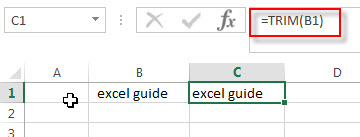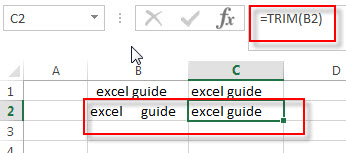# Excel TRIM Function

This post will guide you how to use Excel TRIM function with syntax and examples in Microsoft excel.

## Description

The Excel TRIM function removes all spaces from text string except for single spaces between words.  You can use the TRIM function to remove extra spaces between words in a string. Or removes leading and trailing spaces from the text.

The TRIM function is a build-in function in Microsoft Excel and it is categorized as a Text Function.

The TRIM function is available in Excel 2016, Excel 2013, Excel 2010, Excel 2007, Excel 2003, Excel XP, Excel 2000, Excel 2011 for Mac.

## Syntax

The syntax of the TRIM function is as below:

`= TRIM (text)`

Where the TRIM function argument is:

• Text -This is a required argument. The text string from which you want to remove the extra spaces.

## Excel TRIM Function Examples

The below examples will show you how to use Excel TRIM function to remove extra spaces.

#1 To remove leading spaces in the Cell B1, Just using the following formula:

`=TRIM(B1)`#2 To remove multiple spaces between two words in B2, using the below excel formula:

`=TRIM(B2)`### More Excel TRIM Formula examples

• Split Multiple Lines from a Cell into Rows
If you have multiple lines in a cell and each line is separated by line break character or press “alt + enter” key while you entering the text string into cells, and you need to extract the multiple lines into the separated rows or columns, you can use a combination with the TRIM function, the MID function, the SUBSTITUTE function, the REPT function, the LEN function to create a complex excel formula..…
• Remove Spaces from a String
how to remove extra spaces between words or text string from the specified range of cells in excel. How to delete all spaces from excel cells. …